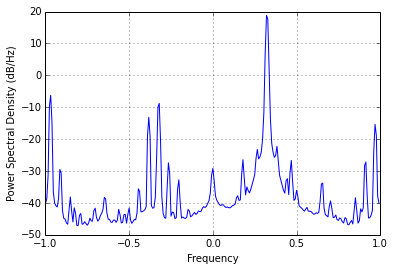In :
filename = "capture.raw"

Fc = 462.4e6    # Center frequency
Fs = 2e6        # Sample rate

N = int(2 * Fs) # Pull in this many samples
gain = 20.0     # Gain

dirname = "/home/jbm/"

In :
from rtlsdr import RtlSdr
%pylab inline

sdr = RtlSdr()

# configure device
sdr.sample_rate = Fs  # Hz
sdr.center_freq = Fc     # Hz
sdr.freq_correction = -52   # PPM
sdr.gain = gain

sdr.close()
del(sdr)

todump = np.array(x).astype("complex64")
todump.tofile(dirname + "/" + filename)

psd(x)

len(x)

Populating the interactive namespace from numpy and matplotlib


Out:
4000000In []: